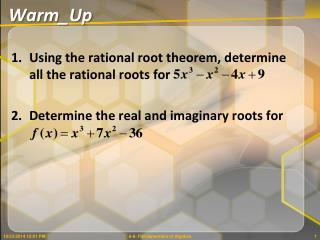DownloadDownload PresentationWarm_Up

Warm_Up

Télécharger la présentationWarm_Up

- - - - - - - - - - - - - - - - - - - - - - - - - - - E N D - - - - - - - - - - - - - - - - - - - - - - - - - - -
Presentation Transcript

1. Warm_Up • Using the rational root theorem, determine all the rational roots for • Determine the real and imaginary roots for 6-6: Fundamentals of Algebra

2. Example 7 Find the real and imaginary rational zeros for 6.5 - Finding Rational Zeros

3. Example 8 Find the real and imaginary rational zeros for 6.5 - Finding Rational Zeros

4. Your Turn Find all real and imaginaryrational zeros for 6.5 - Finding Rational Zeros

5. Fundamental Theorem of Algebra Section 6-6 6-6: Fundamentals of Algebra

6. Review What are the linear functions of 6-6: Fundamentals of Algebra

7. Review Write as the product of linear factors and list all of its zeros. 6-6: Fundamentals of Algebra

8. Fundamental Theorem of Algebra Counting complex and repeated solutions, an nth degree polynomial equation has exactly n solutions. Steps: • Set the problem to equal zero. • Takeout the GCF • Find the factors through Factoring or Quadratic Formula • Set each factor to equal zero and SOLVE. 6-6: Fundamentals of Algebra

9. Example 1 Write the simplest polynomial function with the given zeros of 4, –1, –2 6-6: Fundamentals of Algebra

10. Review Simplify: Conjugate: Remember seeing the sign? 10/23/2014 12:01 PM 6-6: Fundamentals of Algebra 10

11. Writing Functions with Zeros Steps: • Identify all roots. • Square both sides. • Write the equation in factored form. • Multiply to get the polynomial if needed. WORK BACKWARDS! Every radical or imaginary answer given is REALLY TWO ROOTS 6-6: Fundamentals of Algebra

12. Example 3 Write the simplest polynomial function with the given zeros of 6-6: Fundamentals of Algebra

13. Your Turn Write the simplest polynomial function with the given zeros of and 6-6: Fundamentals of Algebra

14. Complex Conjugate Root Theorem Applies to imaginary roots and radicals • Identify all roots. • Take the CONJUGATE ofRADICALSand IMAGINARY NUMBERS **EVERY RADICAL OR IMAGINARY NUMBER HAS TWO ROOTS** • Write the equation in factored form. • Multiply to get the polynomial 6-6: Fundamentals of Algebra

15. Example 4 Write the simplest polynomial function with the given zeros of 2i, , and 1 2 6-6: Fundamentals of Algebra

16. Example 5 Write the simplest polynomial function with the given zeros of –3, ½ and i 10/23/2014 12:01 PM 6-6: Fundamentals of Algebra 16

17. Example 6 Write the simplest function with zeros of 6-6: Fundamentals of Algebra

18. Example 6 Write the simplest function with zeros of 10/23/2014 12:01 PM 18 6-6: Fundamentals of Algebra

19. Example 6 Write the simplest function with zeros of 10/23/2014 12:01 PM 6-6: Fundamentals of Algebra 19

20. Example 7 Write the simplest function with zeros of 10/23/2014 12:01 PM 6-6: Fundamentals of Algebra 20

21. Your Turn Write the simplest function with zeros of 10/23/2014 12:01 PM 6-6: Fundamentals of Algebra 21

22. 1 Vcone = x2h and Vcylinder = x2h . 3 Application A silo is in the shape of a cylinder with a cone-shaped top. The cylinder is 20 feet tall. The height of the cone is 1.5 times the radius. The volume of the silo is 828π cubic feet. Find the radius of the silo. Understand the Problem The cylinder and the cone have the same radius x. The answer will be the value of x. • List the important information: • The cylinder is 20 feet tall. • The height of the cone part is 1.5 times the radius, 1.5x. • The volume of the silo is 828 cubic feet. 6-6: Fundamentals of Algebra

23. V(x) = x3 + 20x2 1 1 2 2 x3 + 20x2 = 828 Application A silo is in the shape of a cylinder with a cone-shaped top. The cylinder is 20 feet tall. The height of the cone is 1.5 times the radius. The volume of the silo is 828π cubic feet. Find the radius of the silo. Write an equation to represent the volume of the body of the silo. V = Vcone + Vcylinder Set the volume equal to 828. 6-6: Fundamentals of Algebra

24. 1 1 1 1 2 2 2 2 6 20 0 –828 x3 + 20x2 – 828 = 0 x3 + 20x2 – 828 = 0 Application A silo is in the shape of a cylinder with a cone-shaped top. The cylinder is 20 feet tall. The height of the cone is 1.5 times the radius. The volume of the silo is 828π cubic feet. Find the radius of the silo. Write in standard form. Divide both sides by . The radius must be a positive number, so the radius of the silo is 6 feet. 3 138 828 23 138 0 6-6: Fundamentals of Algebra

25. Assignment Page 449, 11, 12, 13-21 odd, 25, 27, 31, 35, 36, 39, 42 6-6: Fundamentals of Algebra